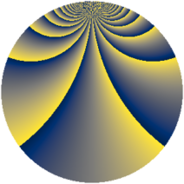Properties

 Label 1089.3.bfLevel $1089$ Weight $3$ Character orbit 1089.bf Rep. character $\chi_{1089}(5,\cdot)$ Character field $\Q(\zeta_{330})$ Dimension $20960$ Sturm bound $396$

Related objects

Defining parameters

 Level: $$N$$ $$=$$ $$1089 = 3^{2} \cdot 11^{2}$$ Weight: $$k$$ $$=$$ $$3$$ Character orbit: $$[\chi]$$ $$=$$ 1089.bf (of order $$330$$ and degree $$80$$) Character conductor: $$\operatorname{cond}(\chi)$$ $$=$$ $$1089$$ Character field: $$\Q(\zeta_{330})$$ Sturm bound: $$396$$

Dimensions

The following table gives the dimensions of various subspaces of $$M_{3}(1089, [\chi])$$.

Total New Old
Modular forms 21280 21280 0
Cusp forms 20960 20960 0
Eisenstein series 320 320 0

Trace form

 $$20960 q - 123 q^{2} - 64 q^{3} + 479 q^{4} - 123 q^{5} - 58 q^{6} - 41 q^{7} - 60 q^{9} + O(q^{10})$$ $$20960 q - 123 q^{2} - 64 q^{3} + 479 q^{4} - 123 q^{5} - 58 q^{6} - 41 q^{7} - 60 q^{9} - 160 q^{10} - 120 q^{11} + 55 q^{12} - 41 q^{13} - 123 q^{14} + 139 q^{15} - 1057 q^{16} - 110 q^{18} - 200 q^{19} - 87 q^{20} - 183 q^{21} - 36 q^{22} - 54 q^{23} - 200 q^{24} + 1229 q^{25} - 67 q^{27} - 116 q^{28} - 42 q^{29} + 308 q^{30} - 41 q^{31} - 12 q^{32} - 167 q^{33} - 76 q^{34} - 518 q^{36} - 164 q^{37} - 1731 q^{38} + 22 q^{39} + 52 q^{40} - 375 q^{41} - 6 q^{42} + 24 q^{43} + 479 q^{45} - 140 q^{46} - 123 q^{47} - 517 q^{48} - 1791 q^{49} - 186 q^{50} + 626 q^{51} + 31 q^{52} + 890 q^{54} - 234 q^{55} - 90 q^{56} - 666 q^{57} + 15 q^{58} + 345 q^{59} + 714 q^{60} - 41 q^{61} - 147 q^{63} - 4044 q^{64} - 360 q^{65} - 726 q^{66} - 120 q^{67} + 126 q^{68} + 332 q^{69} + 110 q^{70} - 1945 q^{72} - 92 q^{73} + 141 q^{74} - 747 q^{75} - 408 q^{76} + 825 q^{77} - 750 q^{78} - 149 q^{79} - 276 q^{81} + 108 q^{82} - 168 q^{83} - 1507 q^{84} + 279 q^{85} - 879 q^{86} - 829 q^{87} + 828 q^{88} + 3038 q^{90} + 1208 q^{91} - 3513 q^{92} + 369 q^{93} + 74 q^{94} - 1281 q^{95} - 1320 q^{96} - 311 q^{97} + 1948 q^{99} + O(q^{100})$$

Decomposition of $$S_{3}^{\mathrm{new}}(1089, [\chi])$$ into newform subspaces

The newforms in this space have not yet been added to the LMFDB.